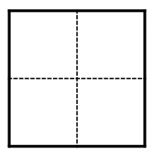시간 제한 메모리 제한 제출 정답 맞은 사람 정답 비율
1 초 32 MB 2 2 2 100.000%

## 문제

Everybody knows that Balázs has the fanciest fence in the whole town. It’s built up from N fancy sections. The sections are rectangles standing closely next to each other on the ground. The ith section has integer height hi and integer width wi.

We are looking for fancy rectangles on this fancy fence.

A rectangle is fancy if:

• its sides are either horizontal or vertical and have integer lengths
• the distance between the rectangle and the ground is integer
• the distance between the rectangle and the left side of the first section is integer
• it’s lying completely on sections

What is the number of fancy rectangles?

This number can be very big, so we are interested in it modulo 109 + 7.

## 입력

The first line contains N, the number of sections.

The second line contains N space-separated integers, the ith number is hi.

The third line contains N space-separated integers, the ith number is wi.

## 출력

You should print a single integer, the number of fancy rectangles modulo 109 + 7. So the output range is 0, 1, 2, . . . , 109 + 6.

## 제한

• 1 ≤ N ≤ 105
• 1 ≤ hi, wi ≤ 109

## 예제 입력 1

2
1 2
1 2


## 예제 출력 1

12


## 힌트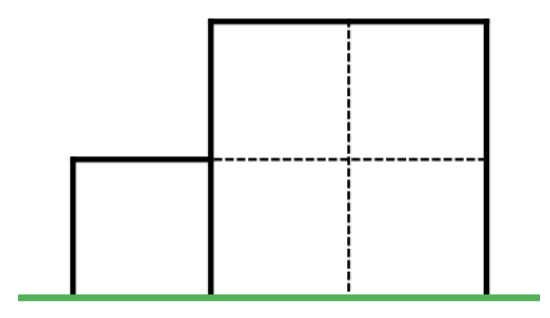There are 5 fancy rectangles of shape: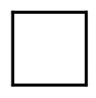There are 3 fancy rectangles of shape: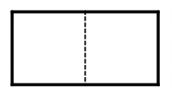There is 1 fancy rectangle of shape: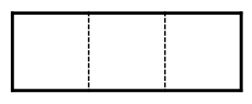There are 2 fancy rectangles of shape: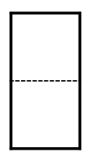There is 1 fancy rectangle of shape: